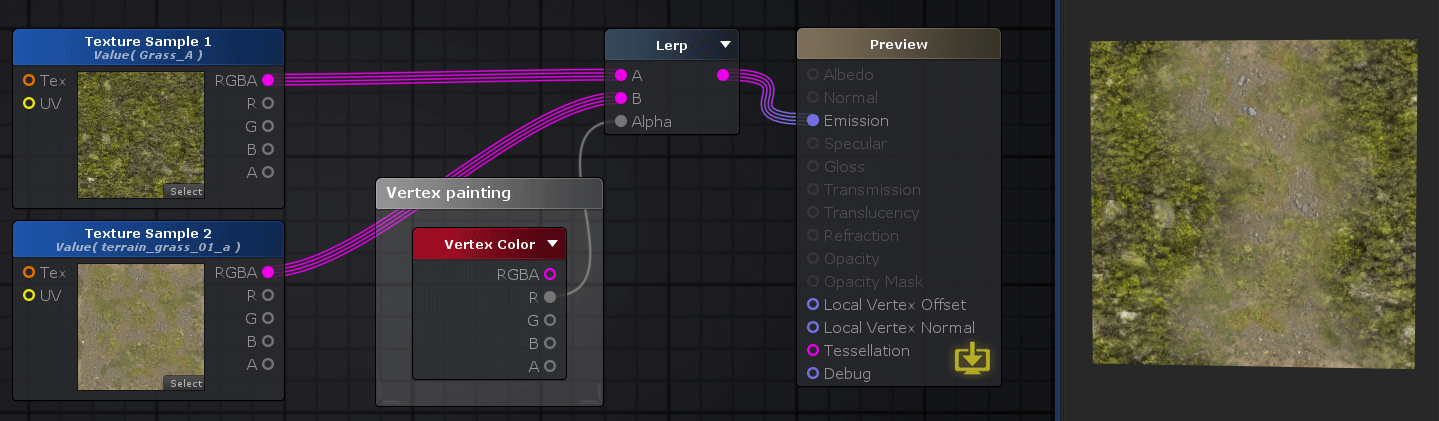# Unity Products:Amplify Shader Editor/Vertex Color

## Vertex Color Node

The Vertex Color node outputs the vertex color specified on the 3D model. An interpolated value is outputted if connected to ports other than Vertex Offset or Vertex Normal.Nodes used: Texture Sample, Vertex Color, Lerp

Output Port Description Type
RGBA Returns the assigned vertex color value Float4
R Returns only the R component of the vertex color value which corresponds to the X component of a Vector4 Float
G Returns only the G component of the vertex color value which corresponds to the Y component of a Vector4 Float
B Returns only the B component of the vertex color value which corresponds to the Z component of a Vector4 Float
A Returns only the A component of the vertex color value which corresponds to the W component of a Vector4 Float